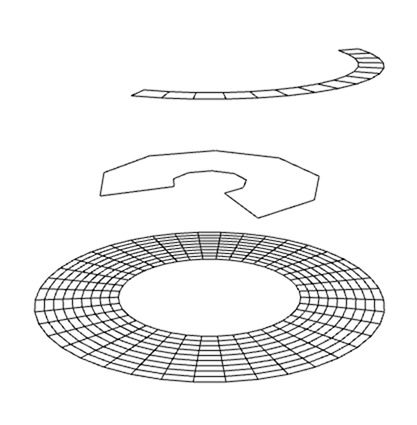# geo-arc

Creates a 2d arc in 3d space

## Usage no npm install needed!

``````<script type="module">
import geoArc from 'https://cdn.skypack.dev/geo-arc';
</script>``````

# geo-arcCreates a 2d arc in 3d space.

## Usage### Example

An example can be found at `./test/index.js`. You can run this test by calling:

``````\$ npm test
``````

A simple usage example with default values being passed as settings:

``````var geoArc = require('geo-arc');

// geo will be a Object will three properties:
// positions - the vertices
// cells - the indices to draw the arc
// uvs - uv values for for the arc
var geo = geoArc( {
cellSize: 3, // 1 == points, 2 == lines, 3 == triangles
x: 0, // x position of the center of the arc
y: 0, // y position of the center of the arc
z: 0, // z position of the center of the arc
Generated uv values look like this: